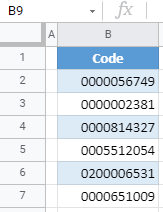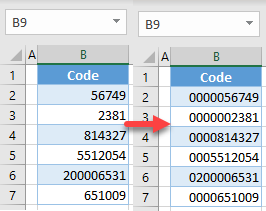## Pad Numbers by Using Custom Cell Format

The first option to pad numbers is to use a custom cell format. Say you have the following list of codes in Column B and each code needs to have 10 digits total, with leading zeros.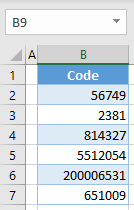The value in B2 needs to start with 5 zeros; B3 needs 6 zeros, etc. To achieve this, follow these steps:

1. Select the range with numbers (B2:B7) and right-click anywhere in the selected area, then choose Format Cells…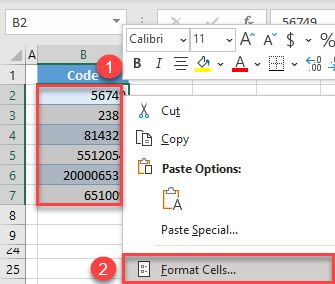1. In the Format Cells window’s Number tab, select Custom in the Category list and enter 0000000000 for Type, then click OK. As you can see in the Sample above Type, Excel adds as many zeros as needed until there are 10 digits.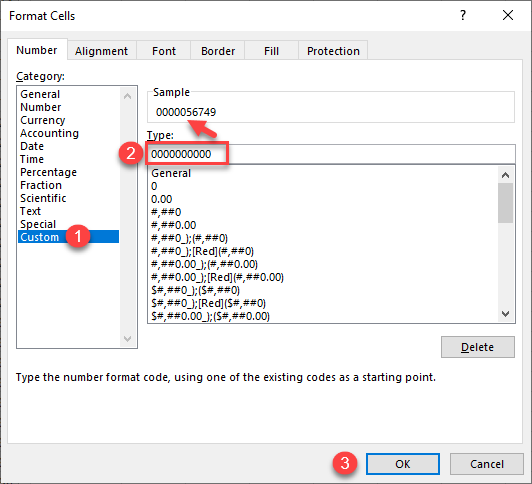Finally, all numbers in the range have a length of 10 with leading zeros.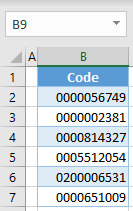Note that these codes are stored as numerical values and only display leading zeros. This means the numbers can be used in calculations, but the zeros are not part of the value and will disappear if the cell format changes.

## Pad Numbers With the TEXT Function

Another option to pad numbers is to use the TEXT Function.

1. Select the adjacent cell (here, C2) and enter this formula:
``=TEXT(B2,"0000000000")``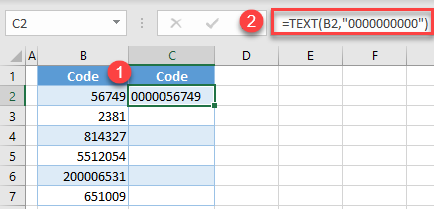1. Drag the formula down the column (through cell C7).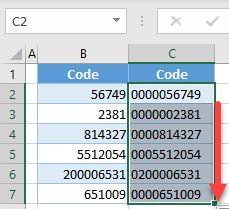Again, all values from Column B have leading zeros. Note that the 10-digit codes are now stored as text, and the leading zeros are a part of the actual value. This means you cannot use these numbers in any calculations, but the characters will be the same regardless of the cell format.

Both custom cell formatting and the TEXT Function can be used in Google Sheets as well. Since the TEXT Function works the same as in Excel, we’ll explain how to pad numbers in Google Sheets using custom formatting.

1. Select the range with numbers (B2:B7). In the Menu, go to Format > Number > More Formats > Custom number format.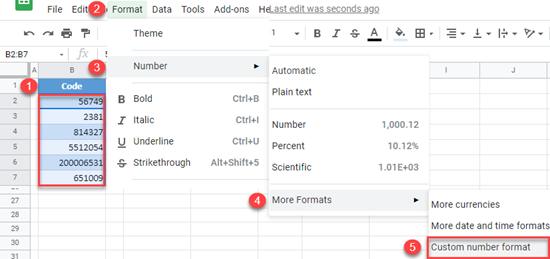1. In the pop-up window, enter 0000000000 and click Apply.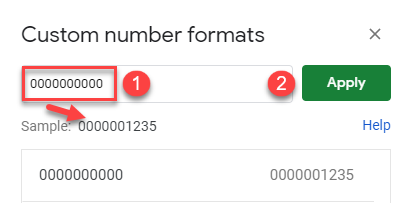The result is the same as in Excel: All codes are displayed as 10-digit numbers.